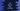# C# program to sort an array## How to sort an array in C#:

To sort an array in C#, we have two predefined methods called Array.Sort. The Sort method can be used to sort an array in different ways.

Let’s take a look at the examples.

### Sorting an array using Array.Sort():

Let’s take a look at the below program:

``````using System;

public class Program {

public static void Main(string[] args) {
int[] arr = {5, 3, 2, 1, 4};

Array.Sort(arr);

Console.WriteLine(string.Join(" ", arr));
}
}``````

In this program, we are sorting the integer array arr. It will print the below output:

``1 2 3 4 5``

### Sorting elements in a range:

We can also pass the start and end indices to sort in a range. Let’s take a look at the below program:

``````using System;

public class Program {

public static void Main(string[] args) {
int[] arr = {5, 3, 2, 1, 4};

Array.Sort(arr, 0, 3);

Console.WriteLine(string.Join(" ", arr));
}
}``````

It will sort all the elements from index 0 to 3. It will print the below output:

``2 3 5 1 4``

As you can see here, the last element is not moved from its position.

### Sort one array based on the values of another array:

Array.Sort can be used to sort elements of one array based on the elements of another array as its key. For example:

``````using System;

public class Program {

public static void Main(string[] args) {
int[] keys = {4, 3, 2, 1};
string[] values = {"Apple", "Orange", "Apricot", "Banana"};

Array.Sort(keys, values);

Console.WriteLine(string.Join(" ", keys));
Console.WriteLine(string.Join(" ", values));
}
}``````

Here,

• keys array is used as the primary array to sort the second array.
• values is the second array and the Sort is used to sort the second array using the first array keys.
• If you run this program, it will print the below output:
``````1 2 3 4
Banana Apricot Orange Apple``````

As you can see here, the first array keys is sorted and the second array values is sorted based on the keys array.

### Sorting an array in reverse order:

We can reverse an array using the Array.Reverse method. This method takes one array as the argument and it reverse the array. We can sort the array and reverse the array to make it inverse sort. For example:

``````using System;

public class Program {

public static void Main(string[] args) {
int[] arr = {4, 3, 2, 1};

Array.Sort(arr);
Array.Reverse(arr);

Console.WriteLine(string.Join(" ", arr));
}
}``````

It will print:

``4 3 2 1``